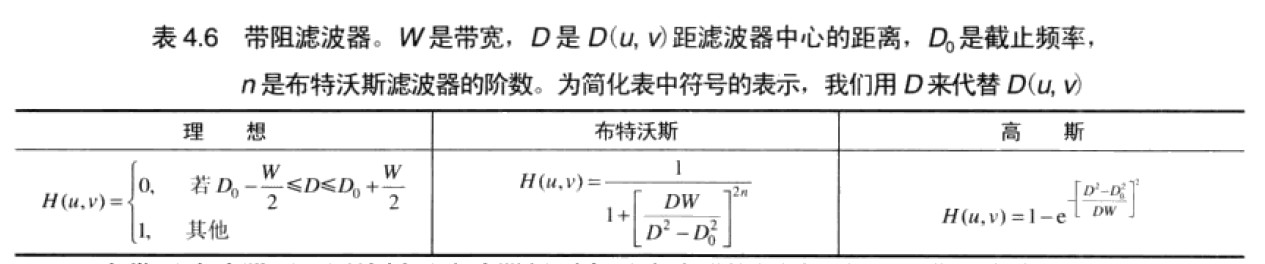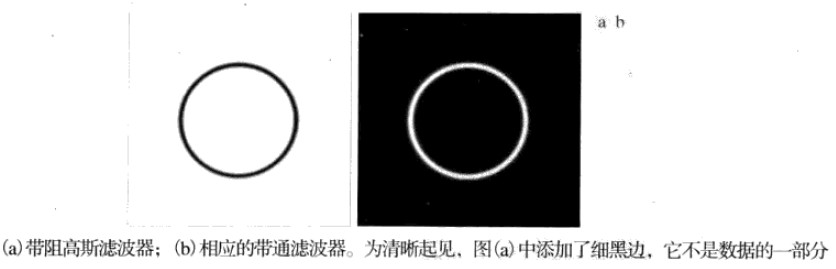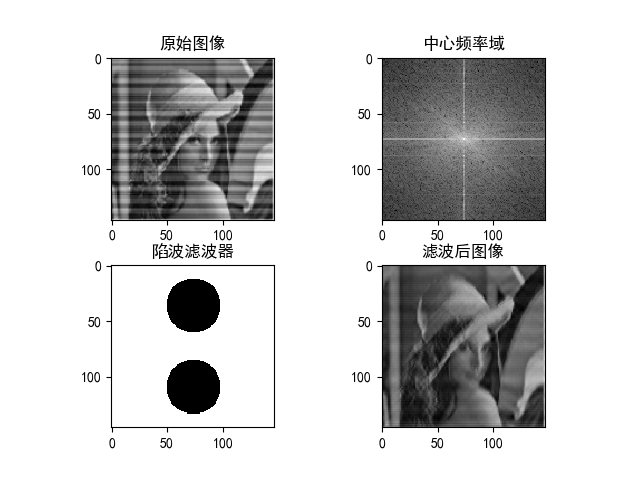2016-11-28 00:47:37 qq_24894159 阅读数 5053
• ###### Qt项目实战之网络电子白板

本课程使用Qt技术实现了网络电子白板，支持直线、矩形、椭圆、三角形、涂鸦等图形元素。本课程实现的电子白板，可以在多人之间共享，每个人都可以进行任意绘制，每个人的绘制都可以同步显示在其它人的白板上。服务器端使用Qt Network开发，客户端使用Qt Network和Qt Graphics View Framework开发，数据传输使用JSON数据格式。

11715 人正在学习 去看看 安晓辉

## 空间域滤波机理

  *空间滤波器由一个邻域（典型的是一个较小的矩形）构成，对该邻域所包围的像素按照一定的操作计算出目标像素的值，这一过程就是空间滤波器的工作机理*


T=111111111## 线性滤波器和非线性滤波器

f(x+y)=f(x)+f(y)f(ax)=af(x)

## 平滑线性滤波器

T1=111111111T2=121242121

function [Image] = fliter (img, type)
%初始化滤波器模板
f = ones(3,3);
[x,y] = size(img);
Image = zeros(x,y);
%注意由于我们是3*3的滤波器，对第一个像素img(1,1)滤波时，滤波器会有
%一部分在图像外部，所以我们要初始一个带着一圈0的壳的图像
temp = zeros(x+2,y+2);
%把中间的部分赋值原来的数值
temp(2:x+1,2:y+1) = img;
for i=2:x+1
for j=2:y+1
%计算均值
Image(i-1,j-1) = mean(mean(f.*temp(i-1:i+1,j-1:j+1))');
end;
end;
endfunction

## 统计排序（非线性）滤波器

### 中值滤波器

function [Image] = medianFliter (img)
[x,y] = size(img);
Image = zeros(x,y);
temp = zeros(x+2,y+2);
%同上面均值滤波的作用
temp(2:x+1,2:y+1) = img;
for i=1:x
for j=1:y
t = temp(i:i+2,j:j+2);
%median为求中值函数，需要将t转化为一个列向量
Image(i,j) = median(t(:));
end;
end;

endfunction## 锐化空间滤波器

### 准备知识

#### 微分我们可以简单的理解为求导fx=f(x+1)f(x)

#### 二阶微分

2f2x=f(x+1)xf(x)x=f(x+1)+f(x1)2f(x)

## 下面我们就正式开始进行图像锐化

### 这里我们采用二阶微分进行锐化先

2f=2fx2+2fy2=f(x+1,y)+f(x1,y)2f(x,y)+f(x,y+1)+f(x,y1)2f(x,y)

T3=010141010

T4=111181111

T5=010141010

T6=111181111

temp(temp<0) = 0;

g(x,y)=f(x,y)+c[2f(x,y)]

function [Image] = sharpen (img)
[x,y] = size(img);
Image = zeros(x,y);
temp = zeros(x+2,y+2);
temp(2:x+1,2:y+1) = img;
%这里我没有采用算子模板进行计算
for i=1:x
for j=1:y
t = temp(i,j+1)+temp(i+2,j+1)+temp(i+1,j)+temp(i+1,j+2)-4*temp(i+1,j+1);
Image(i,j) = t;
end;
end;
Image(Image<0)=0;
figure;
imshow(uint8(Image));
Image = img.-Image;
endfunction### 非锐化掩蔽和高提升滤波

1、模糊化原图。
2、原图减去模糊图像（差值称为模板）。
3、将模板加到原图。
f¯(x,y)$\overline{f}(x,y)$表示模糊图像，

### 使用一阶微分对（非线性）图像锐化——梯度

http://blog.csdn.net/ubunfans/article/details/42214925

Good Night！

2015-07-27 17:38:18 xuhang0910 阅读数 2363
• ###### Qt项目实战之网络电子白板

本课程使用Qt技术实现了网络电子白板，支持直线、矩形、椭圆、三角形、涂鸦等图形元素。本课程实现的电子白板，可以在多人之间共享，每个人都可以进行任意绘制，每个人的绘制都可以同步显示在其它人的白板上。服务器端使用Qt Network开发，客户端使用Qt Network和Qt Graphics View Framework开发，数据传输使用JSON数据格式。

11715 人正在学习 去看看 安晓辉

# 1.低通滤波器

//使用低通滤波器
#include<opencv2\opencv.hpp>
#include<iostream>

using namespace cv;
using namespace std;

int main()
{
Mat dst;
Mat dstG;
cv::blur(src, dst, cv::Size(7, 7));//Size（7，7）表示的相邻像素的范围，数字越大越模糊。

imshow("car", src);
imshow("car2", dst);

waitKey(50000);

return 0;
}# 2.中值滤波器mediaBlur

//中值滤波器medianBlur
#include<opencv2\opencv.hpp>
#include<iostream>
using namespace cv;

int main()
{
Mat dst;

cv::medianBlur(src, dst, 5);

imshow("car2",dst);
imshow("car", src);
waitKey(20000);

return 0;
}# 3.Sobel滤波

//使用Sobel滤波器
#include<opencv2\opencv.hpp>
#include<iostream>

using namespace cv;
using namespace std;

int main()
{
Mat sobelX;
Mat sobelY;
//对X方向求导，sobelX保留了垂直方向的信息，所以垂直的轮廓看起来更清楚
cv::Sobel(src,sobelX, CV_8U, 1, 0, 3, 0.4, 128);
//检测垂直方向的边缘
cv::Sobel(src, sobelY, CV_8U, 0, 1, 3, 0.4, 128);

imshow("car", src);
imshow("sobelX", sobelX);
imshow("sobelY", sobelY);

waitKey(50000);

return 0;
}//使用Sobel滤波器
#include<opencv2\opencv.hpp>
#include<iostream>
#include<opencv2\imgproc\imgproc.hpp>

using namespace cv;
using namespace std;

int main()
{
Mat sobelX;
Mat sobelY;
Mat sobel;
cv::Sobel(src,sobelX, CV_8U, 1, 0, 3, 0.4, 128);
cv::Sobel(src, sobelY, CV_8U, 0, 1, 3, 0.4, 128);
//合并结果
sobel = abs(sobelX) + abs(sobelY);
//搜索Sobel极大值
double sobmin, sobmax;
cv::minMaxLoc(sobel, &sobmin, &sobmax);
//变换为8位图像
Mat sobelImage;
sobel.convertTo(sobelImage, CV_8U, -255. / sobmax, 255);
//阈值化处理，得到二值图像
Mat sobelThresholded;
cv::threshold(sobelImage, sobelThresholded,70, 255, cv::THRESH_BINARY);

imshow("car", sobelThresholded);
waitKey(50000);

return 0;
}好像结果不太理想

2011-12-31 21:35:00 kanyun157 阅读数 22
• ###### Qt项目实战之网络电子白板

本课程使用Qt技术实现了网络电子白板，支持直线、矩形、椭圆、三角形、涂鸦等图形元素。本课程实现的电子白板，可以在多人之间共享，每个人都可以进行任意绘制，每个人的绘制都可以同步显示在其它人的白板上。服务器端使用Qt Network开发，客户端使用Qt Network和Qt Graphics View Framework开发，数据传输使用JSON数据格式。

11715 人正在学习 去看看 安晓辉

对每个窗口都要求和，然后求均值，而且是对每个像素点进行遍历，当然是个费时的过程。一般的操作可能是这样的：

sum=0;
for(i=0;i<w;i++)
{
for(j=0;j<w;j++)
{
sum+=Array[m+i][n+j];
}
}
mean=sum/(w*w);

for(m=0;m<m_Height;m++)
{
for(n=0;n<m_Width;n++)
{
if(n==0)
{
sum=0;
for(i=0;i<w;i++)
{
for(j=0;j<w;j++)
{
sum+=Array[m+i][n+j];
}
}
}
else
{
a=b=0;
for(i=0;i<w;i++)
{
a+=Array[m+i][n+w-1];
b+=Array[m+i][n-1];
}
sum=sum+a-b;
}
mean=sum/(w*w);

｝

｝

2017-09-25 16:56:33 wang2012010865 阅读数 471
• ###### Qt项目实战之网络电子白板

本课程使用Qt技术实现了网络电子白板，支持直线、矩形、椭圆、三角形、涂鸦等图形元素。本课程实现的电子白板，可以在多人之间共享，每个人都可以进行任意绘制，每个人的绘制都可以同步显示在其它人的白板上。服务器端使用Qt Network开发，客户端使用Qt Network和Qt Graphics View Framework开发，数据传输使用JSON数据格式。

11715 人正在学习 去看看 安晓辉（1-1）（1-2）（1-3）（1-4）

（1）在恒定灰度区域的微分值为零；（2）在灰度台阶或者斜坡处微分值非零；（3）沿着斜坡或者台阶的微分值非零。

（1）在恒定区域微分值为零；（2）在灰度台阶或者斜坡起点处微分值为零；（3）沿着斜坡的微分值非零。（1-5）（1-6）（1-7）（1-8）（1-9）（1-10）

（1）模糊原图像。
（2）从原图像中减去模糊图像（产生的差值图像称为模板）。
（3）将模板加到原图像上。（1-11）（1-12）（1-12）（1-13）（1-14）（1-15）（1-16）（1-17）

>（1-18）（1-19）（1-20）
（1-18）和（1-19）所代表的算子称为Sobel算子。其中模板所有系数和为零。

2018-12-17 17:30:45 HHH_ANS 阅读数 836
• ###### Qt项目实战之网络电子白板

本课程使用Qt技术实现了网络电子白板，支持直线、矩形、椭圆、三角形、涂鸦等图形元素。本课程实现的电子白板，可以在多人之间共享，每个人都可以进行任意绘制，每个人的绘制都可以同步显示在其它人的白板上。服务器端使用Qt Network开发，客户端使用Qt Network和Qt Graphics View Framework开发，数据传输使用JSON数据格式。

11715 人正在学习 去看看 安晓辉

# 1 - 引言

1. 带阻滤波器或带通滤波器
2. 陷波滤波器
下面就让我们来介绍一下这两种滤波器

# 2 - 带阻滤波器和带通滤波器# 3 - 陷波滤波器

$H_{HR}(u,v)=\prod_{k=1}^QH_k(u,v)H_{-k}(u,v)$

$D_k(u,v)=[(u-M/2-u_k)^2+(v-N/2-v_k)^2]^{1/2}$

$D_{-k}(u,v)=[(u-M/2+U_k)^2+(v-N/2+v_k)^2]^{1/2}$

$H_{NR}(u,v)=\prod_{k=1}^2[\frac{1}{1+[D_{0k}/D_k(u,v)^{2n}]}][\frac{1}{1+[D_{0k}/D_{-k}(u,v)^{2n}]}]$

import cv2
import numpy as np
import matplotlib.pyplot as plt

#解决中文显示问题
plt.rcParams['font.sans-serif']=['SimHei']
plt.rcParams['axes.unicode_minus'] = False

f = np.fft.fft2(img)
fshift = np.fft.fftshift(f)
s1 = np.log(np.abs(fshift))
plt.subplot(221),plt.imshow(img,'gray'),plt.title('原始图像')
plt.subplot(222),plt.imshow(s1,'gray'),plt.title('中心频率域')

w , h = img.shape
"""设计理想陷波滤波器"""
flt = np.zeros(img.shape)
rx1 = w / 4
ry1 = h / 2

rx2 = w*3/4
ry2 = h/2;

r = min(w,h)/6 #半径
for i in range(1,w):
for j in range(1,h):
if ((i - rx1)**2 + (j - ry1)**2 >= r**2) and ((i - rx2)**2 + (j - ry2)**2 >= r**2):
flt[i,j] = 1

plt.subplot(223),plt.imshow(flt,'gray'),plt.title('陷波滤波器')
new_img = np.abs(np.fft.ifft2(np.fft.ifftshift(fshift*flt)))
plt.subplot(224),plt.imshow(new_img,'gray'),plt.title('滤波后图像')

plt.show()